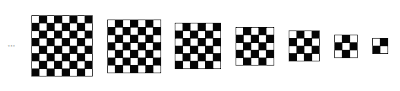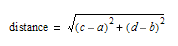Technology for Artists

A discussion of ... uh, technology for artists. Duh!

Wednesday, September 19, 2007

Math Without Math: Distances

I'll go back to rasters and vectors, but I wanted to talk about one more idea on Cartesian coordinates, basically, on thinking of a sheet of paper (or any surface) as a grid of rows and columns.

If we make the rows and columns the same size, like inches or centimeters, then we can measure distances just by knowing where two points are. The simple case is if you have two points that are on the same horizontal or vertical line. For example, suppose two points are both 1 inch from the bottom of a page. One point is 2 inches from the left edge, and the other is 7 inches from the left edge. You can write this as (2,1) and (7,1), meaning two inches from the left and one inch from the bottom, or seven inches from the left and one inch from the bottom. Then the distance between them is 7 - 2, or 5 inches. (C’mon, you can handle a little subtraction, can't you?)

The harder case is if the two points are not on a horizontal or vertical line. Then you need a little bit of math called the Pythagorean Theorem. If one point is 4 inches from the left and 7 inches from the bottom (i.e., (4, 7)), and the other is 7 inches from the left and 3 inches from the bottom (7, 3), then the distance is five inches. How do we know that? Here are two methods:

The math way ...The non-math way ...
Get a ruler.

But if you want the gritty details, which would entitle you to say Pythagorean Theorem to your friends, you need to know what a square root is. The square of a number is that number multiplied by itself. So 25 is the square of 5 (i.e., 5 × 5), 16 is the square of 4 (4 × 4), etc. The square root is the reverse of that. It's the number which, when multiplied by itself, equals the original number. The square root of 25 is 5, the square root of 16 is 4, etc.

One way to think of this is to imagine you have a checkerboard with 64 squares in it. Then each side of the checkerboard would be 8 squares long. So 8 squared is 64, and the square root of 64 is 8. Likewise for a 7 × 7 (49 square) checkerboard, and for 9 × 9 (81 squares), 5 × 5 (25 squares), and so on for larger numbers and for smaller numbers.The Pythagorean Theorem says that to find the distance between two points, first find the horizontal and vertical distances, square them, add the results, and then take the square root of that. So if the two points were (a, b) and (c, d), then the distance would beNow I know that looks a lot like math, so let's unravel it to see what it's really saying. Let's look at the picture to start.Basically, what Pythagoras noticed was that if you make a square from each side of a right triangle, the two smaller squares add up to the larger square. In our example, we're trying to find the distance between two points. The first point is the top corner of the triangle, at (6, 8). (Just count the boxes, starting at the lower left corner. The second point is the bottom right corner of the triangle, at (9, 4). So the length of the bottom side of the triangle is 3 (which is 9-6), and the left side is 4 (8-4). However, it's not so easy to find the length of the third side, which is the distance we want. You can't just count the boxes.

If you square those lengths, you get 32=9 for the bottom side, and 42=16 for the left side. Adding 9+16=25, which happens to be 52. So the length of the long diagonal side is 5.

I purposely picked a triangle with sides 3, 4 and 5 to make the numbers come out simple. I could, for example, have used 4 and 7 for the short sides, but then the diagonal would be 8.0622577.... The Pythagorean Theorem holds for any right triangle. A right triangle is a triangle that has one corner which is a right, or square, angle. You can see in the diagram above the bottom left corner is a right angle.

If you want some math jargon to sling around, a corner of a triangle is called a vertex, and the long side of a right triangle is called the hypotenuse. Remember that scene in The Wizard of Oz where the Scarecrow gets a diploma and suddenly realizes that "the square of the hypotenuse is equal to the sum of the squares of the other two sides?" That's the Pythagorean Theorem. Now you too can be a Doctor of Thinkology!

In math, a theorem is an idea that seems to be true, but hasn't been proven. So the Pythagorean Theorem hasn't had a rigorous mathematical proof yet. However, keep in mind that Pythagoras came up with this idea over 2500 years ago, and no one has found a single case where it doesn't work, so it's pretty safe.

1 comment:Anonymous said...

Dear Author www.technologyforartists.com !
Earlier I thought differently, many thanks for the information.